# Class 7 Maths NCERT Solutions for Chapter – 4 Simple Equations EX – 4.4

## Simple Equations

Question 1.
Set up equations and solve them to find the unknown numbers in the following cases :
(a)
Add 4 to eight times a number; you get 60.
(b) One fifth of a number minus 4 gives 3.
(c) If I take three fourths of a number and add 3 to it, I get 21.
(d) When I subtracted 11 from twice a number, the result was 15.
(e) Munna subtracts thrice the number of notebooks he has from 50, he finds the result to be 8.
(f) Bengal thinks of a number. If she adds 19 to it and divides the sum by 5, she will get 8.
(g) Anwar thinks of a number. If he takes away 7 from$\frac { 5 }{ 2 }$ of the number, the result is 23.

Solution:
(a) Let x be the required number. Then, the required equation is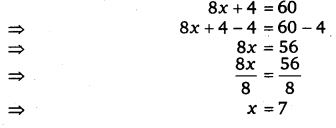(b) Let x be the required number. Then, the required equation is$\frac { x }{ 5 }$ – 4 = 3.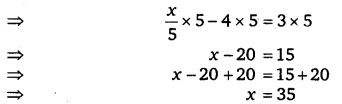(c) Let y be the required number. Then, the required equation be$\frac { 3y }{ 4 }$ + 3 = 21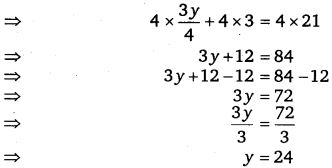(d) Let the required number be m. Then, the required equation is 2m -11 = 15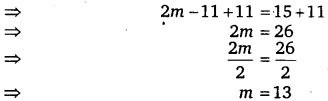(e) Let Munna have x notebooks. Then, the required equation is 50 – 3x = 8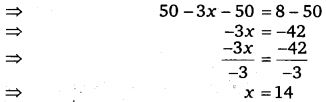(f) Let the number be x. Then, the required equation is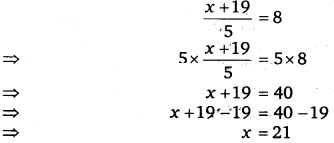(g) Let the number be n. Then, the required equation is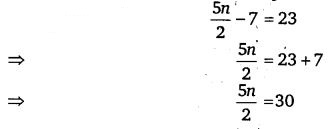Question 2.
Solve the following :

(a) The teacher tells the class that the highest marks obtained by a student in her class is twice the lowest marks plus 7. The highest score is 87. What is the lowest score?

(b) In an isosceles triangle, the base angles are equal. The vertex angle is 40°. What are the base angles of the triangle? (Remember, the sum of three angles of a triangle is 180°).

(c) Sachin scored twice as many runs as Rahul. Together, their runs fell two short of a double century. How many runs did each one score?

Solution:

Question 3.
Solve the following :
(i)
Irfan says that he has 7 marbles more than five times the marbles Pannit has. Irfan has 37 marbles. How many marbles does Permit have?
(ii) Laxmi’s father is 49 years old. He is 4 years older than three times Laxmi’s age. What is Laxmi’s age?
(iii) People of Sundargram planted trees in the village garden. Some of the trees were fruit trees. The number of non-fruit trees were two more than three times the number of fruit trees. What was the number of fruit tree planted if the number of non-fruit trees planted was 77?

Solution:

Question 4.
Solve the following riddle :
I am a number,
Tell my identity!
Take me seven times over